### Prior Distributions

The following list displays the parameterization of prior distributions for situations in which the FMM procedure uses a conjugate sampler in mixture models without model effects and certain basic distributions (binary, binomial, exponential, Poisson, normal, and). You specify the parametersandin the formulas below in the MUPRIORPARMS and PHIPRIORPARMS options in the BAYES statement in these models.

Beta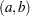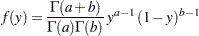where
,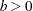. In this parameterization, the mean and variance of the distribution are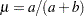and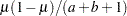, respectively. The beta distribution is the prior distribution for the success probability in binary and binomial distributions when conjugate sampling is used.

Dirichlet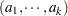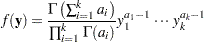where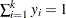and the parameters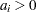. If any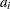were zero, an improper density would result. The Dirichlet density is the prior distribution for the mixture probabilities. You can affect the choice of thethrough the MIXPRIORPARMS option in the BAYES statement. If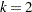, the Dirichlet is the same as the betadistribution.

Gamma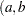)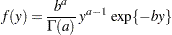where,. In this parameterization, the mean and variance of the distribution are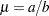and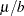, respectively. The gamma distribution is the prior distribution for the mean parameter of the Poisson distribution when conjugate sampling is used.

Inverse gamma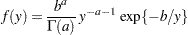where,. In this parameterization, the mean and variance of the distribution are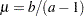if
and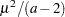if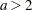, respectively. The inverse gamma distribution is the prior distribution for the mean parameter of the exponential distribution when conjugate sampling is used. It is also the prior distribution for the scale parameter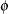in all models.

Multinomial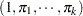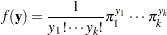where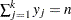,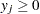,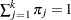, andis the number of observations included in the analysis. The multinomial density is the prior distribution for the mixture proportions. The mean and variance of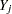are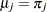and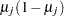, respectively.

Normal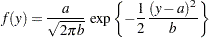where. The mean and variance of the distribution are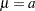and, respectively. The normal distribution is the prior distribution for the mean parameter of the normal anddistribution when conjugate sampling is used.

When a MODEL statement contains effects or if you specify the METROPOLIS option, the prior distribution for the regression parameters is multivariate normal, and you can specify the means and variances of the parameters in the BETAPRIORPARMS option in the BAYES statement.